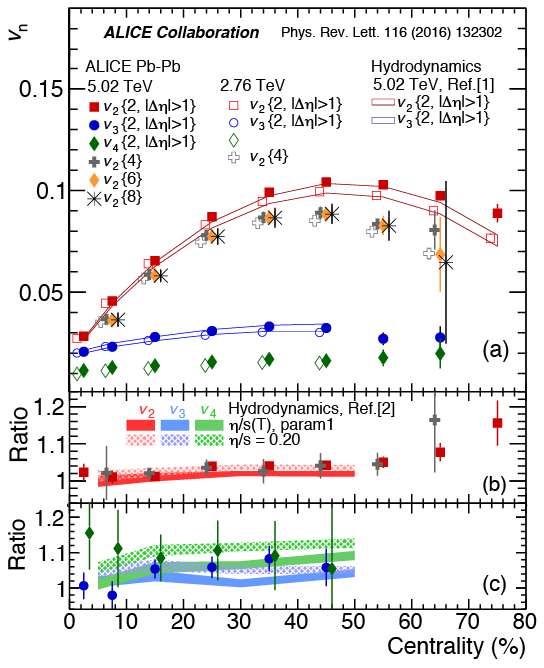### A perfect liquid at the LHCOff-centre nuclear collisions, with a finite impact parameter, create a strongly asymmetric "almond-shaped" fireball. With measuring the momentum distributions of the emitted particles, precious information is obtained.The measured azimuthal momentum distribution of particles emitted from the decaying fireball and the initial spatial asymmetry are correlated due to the multiple interactions between the constituents of the created matter; in other words, the correlation tells us about how the matter expands and flows, which is related to its equation of state of nuclear matter (similarly to the equation of state of water from ice to liquid then to steam with increasing temperature) and its thermodynamic transport properties.The measured azimuthal distribution of particles in momentum space can be decomposed into Fourier coefficients (vn with n=1, 2, 3…). These Fourier coefficients (vn), defining the anisotropic flow, are particularly sensitive to the internal friction or viscosity of the fluid, or more precisely, η/s, the ratio of the shear viscosity (η) to entropy density (s) of the medium. For a good fluid such as water, the η/s ratio is small whereas a "thick" liquid, such as honey, has large values of η/s. The value of η/s which was inferred is close to the lowest limit stipulated by theoretical calculations: this leads to dubbing the Quark-Gluon Plasma “a perfect liquid”.With these measurements for different colliding systems (for instance Pb-Pb or Xe-Xe) and selecting collisions from the peripheral to the most central ones, ALICE is precisely studying the properties of the Quark-Gluon Plasma and the way it can expand and cool down. One important aspect is to explore the temperature dependence of η/s and many in-depth flow-related measurements at the LHC. Such information will further constrain the hydrodynamic features of the Quark-Gluon Plasma.